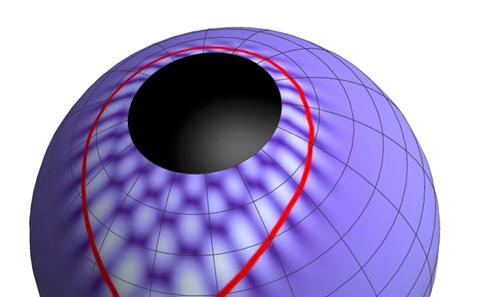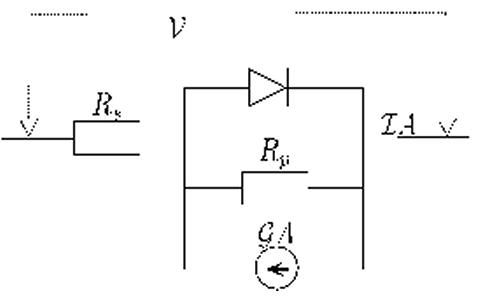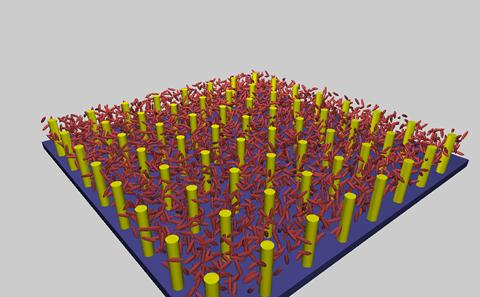The University of Southampton
We're launching a new website soon and would love your feedback. See the new design
Mathematical Sciences

# Research project: Asymptotic Methods

Currently Active:
Yes

Our studies in this field focus on two areas. The first is exponential asymptotics, i.e. how exponentially small terms that are usually neglected, may become dominant and determine the mathematical and physical behaviour of the system under study. The second is multiscale methods: the aim of these methods is to obtain equations for the macroscopic properties of a material or device, that incorporate in a rigorous way, the properties of if microstructure.## Exponential asymptotics

Accurate approximations can often be derived by expanding a function in terms of a small (or large) asymptotic parameter involved in the problem. Often such series diverge for any value of the asymptotic parameter. Poincaré's definition of an asymptotic expansion provided a calculus for their rigorous treatment. However, his definition ignores exponentially smaller scales which are fundamental for resolving ambiguities as well as controlling the divergence and range of validity of the series. Exponential asymptotics addresses all these problems. Rooted in the theory of resurgence, it exploits universal relationships between the components of asymptotic expansions to incorporate the missing exponentially small terms with little extra effort. The mathematical successes of this extremely powerful approach include: exact, remainder terms for wide classes of expansions; improved rigorous error bounds; hyper-exponential improvement in numerical approximations; systematic calculation of Stokes constants; geometric information from spectral functions; long time-asymptotics of nonlinear PDEs; higher-order Stokes phenomena taking place at sub-sub-dominant scales; solvability conditions for PDE problems. Notable applications to physical systems include the explanation of why smoothed nonlinear shocks are not caustics, the discovery of forward beaming of noise from sources in the wake of jet engine flow as well as to string theory. Southampton is a key centre for research in exponential asymptotics with active collaborations in the US, UK, Europe, Asia and Australia.## Multiscale asymptotics

A variety of different models are often used to describe the same physical phenomena on different lengthscales. For example, an experimental physicist might choose to describe current flowing through a solar cell in terms of the equivalent circuit pictured above. However a more fundamental understanding of the same cell would come from considering the flow of electrons and holes in the semiconductors from which its constructed. Whilst the experimentalist knows that they can get a good fit to the current-voltage data measured from a particular cell, by calibrating the components of the equivalent circuit correctly, they might also like to know how modifications to cell construction (e.g. choice of semiconductor and contact materials) affect its performance. Asymptotic methods can be used to show that the gross features of a mesoscopic description of the cell, provided by a charge transport model for electrons and holes, are well approximated by an equivalent circuit model and can be used to determine the properties of its components in terms of the cells construction. Such methods can be used to systematically derive simplified models from complex ones in a wide variety of applications ranging from superconducting vortex motion to the electrical activity of heart.## Homogenisation

Many physical large-scale physical systems are formed by the repetition of many smaller parts (cells) that vary only slightly one from the other. For example, a crystal is formed by the identical repetition of a group of atoms (the unit cell of the crystal lattice). The picture above shows a schematic representation of liquid crystal molecules (red ellipsoid) in a periodic lattice of gold pillars. Modelling systems such as these is computationally expensive, because we need to keep track both of the small scale of the cell and of the large scale of the device. Homogenisation is a rigorous mathematical tool to obtain macroscopic equations, i.e. equations on the length scale of the device, whose parameters depend in a precise and computable manner, on the properties of the system at the unit cell length-scale. We use this technique to model liquid crystals doped with nanoparticles. For example, we have shown that the presence of fixed inclusions is equivalent to adding an additional force field that orients the liquid crystal molecules.

### Related research groups

Applied Mathematics and Theoretical Physics
Computational Applied Mathematics